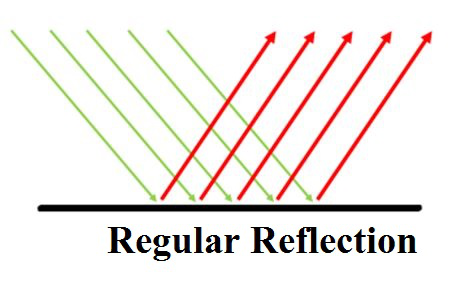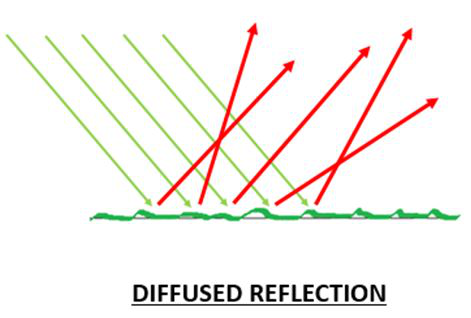GFG App
Open AppBrowser
Continue

# Regular and Diffused Reflection

The light gets reflected from the surfaces. Any surface which is polished or in other words is shiny always acts like a mirror. The observation of light bouncing off the surfaces is termed reflection. The light after reflection travels in the same medium from where the ray was incident on the surface. The phenomenon of reflection doesn’t change the velocity of light it only reverses the direction of light incident on it. This can be observed on any surface which is rough or smooth. The path of the reflected ray will depend upon the extent of smoothness of the surface, in the case of a smooth surface the reflected ray emerges with the same angle as of incidence and in the latter case suffers irregular reflection and so the reflected ray doesn’t emerge same as that of incidence angle.

### Laws of Reflection

1. The ray of light that falls on a reflecting surface is known as the incident ray and the ray that is reflected back is known as the reflected ray.
2. An imaginary line that is perpendicular to the reflecting surface from where the reflection occurs is known as the normal.
3. The angle of incidence ∠i refers to the angle between the incident rays with the normal. The angle of reflection ∠r refers to the angle between the reflected rays with the normal.

There are two types of reflection one is Regular and the second is Diffused reflection.

### Regular Reflection

The bouncing back of the incident light from the surface is known as reflection. If the reflection occurs following the laws of reflection it is known as the regular reflection. It follows both the laws of reflection. The reflected ray from the surface forms the same angle of reflection which is equal to the angle of incidence. The incident ray, reflected ray, and normal which are at the point of incidence, all three of them lie in the same plane. This is observed in the case of polished surfaces which have a shiny appearance such as mirrors.### Diffused Reflection

The going back of the incident light from the surface is known as reflection. In the case of diffused reflection, the reflection doesn’t occur following the laws of reflection. It doesn’t follow any of the laws of reflection. The reflected ray from the surface doesn’t form the same angle of reflection which is equal to the angle of incidence. Also, the incident ray, reflected ray, and normal which is at the point of incidence, all three of them do not lie in the same plane. This is observed in the case of unpolished or rough surfaces like that of paper, cardboard, sandpaper, rough sheets, etc.The following table gives the difference between the regular and diffused reflection:

### Sample Problems on Reflection

Question 1: What will be the angle of reflection for the incident angle 60°?

Solution:

We know that,

∠i = ∠r

Therefore, ∠r = 60°

Hence, the angle of reflection will be 60°.

Question 2: What will be the angle of incidence for the reflected angle 50°?

Solution:

We know that,

∠i = ∠r

Therefore, ∠i = 50°

Hence, the angle of reflection will be 50°.

Question 3: What will be the incident angle if the angle between the mirror surface and the incident ray is 60°?

Solution:

We know that,

∠i + angle between mirror and incident ray = 90°

Therefore, ∠i + 60° = 90°

Therefore, ∠i = 90° – 60°

Therefore, ∠i = 30°

Hence, the incident angle is 30°

Question 3: What will happen if the incident ray falls along the normal into the mirror surface?

We know,

∠i = ∠r

If the incident ray fall along the normal then the angle between the incident ray and the normal becomes zero hence, the reflected angle also becomes zero therefore it means that the light doesn’t experience any reflection and travels straight from the surface.

Question 4: Write two characteristics of diffused reflection.

The two characteristics of diffused reflection are:

1. Reflected rays are not parallel to each other.
2. Reflection cannot be observed clearly.

Question 5: State two laws of reflection.